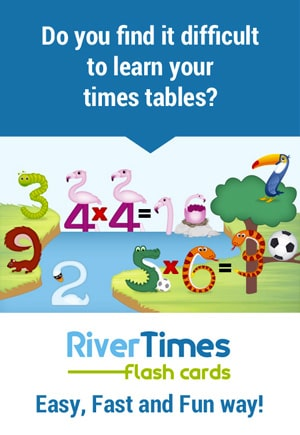# Free & Printable multiplication Charts (14)

2 Reviews

We offer you many versions of printable multiplication Charts completely free-of-charge. These resources will help you learn or revise your times tables

• Chart of different sizes: Choose the one that suits your level: Chart 1-10 (10x10), Chart 1-12 (12x12) or Chart 1-20 (20x20).
• Complete Charts or Charts to be filled out: Complete charts are intended for learning, while blank chart are ideal for revision.
• Colorful or black and white Charts: A colorful chart will help you to memorise more easily. You can also choose to color a black and white table yourself.

Find below our advice and tricks to make the learning easier for you. If you are just starting out, please read How to read a multiplication Chart?

## How to read a multiplication Chart?

Example: What is the result of 3 x 4 multiplication? ?

• 1- Locate the first number in the first column of the chart (number 3 in this example)
• 2- Locate the second number in the first line of the chart (number 4 in this example)
• 3- The square corresponding to the intersection between the line of the first number and the column of the second number contains the result of the multiplication (12 in this example)

## Our tricks for learning your multiplication Chart

### Bring colour into your Chart!

You can print a multiplication Chart already colored or a Multiplication Chart black/white that you will color yourself using your favourite colors! Indeed, the color will make it easier for you to remember thanks to the visual memory process. So, let's get your felt pens!

If you decide to color your chart yourself using your own color codes, you must assign a different colour to each number.
For example: If you assign the green to the number 3, you will have to color in green the following squares: 3x3, 3x4, 3x5, 3x6, 3x7, 3x8, 3x9 (horizontal line) as well as 4x3, 5x3, 6x3, 7x3, 8x3, 9x3 (vertical line). 3x2, belongs to the 2 times table, so it will have to be colored with the color of the 2 times table. Indeed, the result of 3x2 is equal to the result of 2x3, it is therefore useless to learn both, when you know the first, you find the second.
Other example: If you assign the pink to the number 4, you will have to color in pink the following squares: 4x4, 4x5, 4x6, 4x7, 4x8, 4x9 as well as 5x4, 6x4, 7x4, 8x4, 9x4, and so on...
Note: Don't color the squares corresponding to the 1 and 10 times table because the results are very easy and you will not need to learn them by heart!

### No need to learn the whole multiplication Chart

A chart 1-10 (or 10x10), contains 100 squares, so 100 results, it may seem like a lot, but... Great news! You will see that there are many squares that you do not need to learn :) In fact, there are only 36 multiplications to be known. Find below the results of the multiplication chart that you don't need to learn.

#### 1- Don't learn duplicates:

There are many equivalent multiplications in a chart. Indeed, in a multiplication operation, you can invert the numbers while obtaining the same result..
For example: 7 x 6 = 6 x 7 = 42
So if you know the result of 6 x 7, you also know the result of 7 x 6!

Once all duplicates have been removed, there are only 55 multiplications left to learn.

#### 2- No need to learn by heart the 1 and 10 times table:

The 1 times table and the 10 times table are the 2 easiest, it is therefore useless to learn them by heart.
1 times Table: A number multiplied by 1 is always equal to the number.
Example: 1x1=1, 1x2=2, 1x3=3, 1x4=4...
10 times Table: A number multiplied by 10 is always equal to the number followed by a zero.
Example: 1x10=10, 2x10=20, 3x10=30, 4x10=40...

So you can see that, in fact, there are only 36 multiplications to learn . That's pretty good news, isn't it?

### Above all, do not neglect revising your Times tables

The revision of the multiplication table is a necessary step for a long term memorization, so don't neglect it! There are several ways to revise your times tables:

#### 1- Fill in a Blank multiplication Chart:

Completing a blank multiplication chart is an excellent way to revise your tables. At the top of this page, you will find different versions of blank printable blank charts to make revisions easier for you.

#### 2- Use our printable multiplication Worksheets:

Worksheets are also an effective way to revise your tables. You will find several worksheets to make revision easier in our printable multiplication Worksheets section.

### Need a boost to memorize the times tables?

If you have trouble memorizing some of (or even all) the multiplications, discover our Method to Easily learn times tables with the RiverTimes Flash cards, an Original, Fast and Fun way!